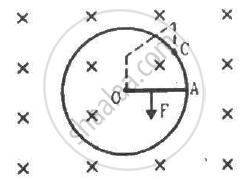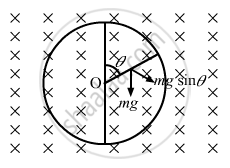Advertisement Remove all ads

# Consider the Situation Shown in the Figure. Suppose the Circular Loop Lies in a Vertical Plane. the Rod Has a Mass M. - Physics

Sum

Consider the situation shown in the figure. Suppose the circular loop lies in a vertical plane. The rod has a mass m. The rod and the loop have negligible resistances but the wire connecting O and C has a resistance R. The rod is made to rotate with a uniform angular velocity ω in the clockwise direction by applying a force at the midpoint of OA in a direction perpendicular to it. Find the magnitude of this force when the rod makes an angle θ with the vertical.Advertisement Remove all ads

#### Solution

When the circular loop is in the vertical plane, it tends to rotate in the clockwise direction because of its weight.Let the force applied be F and its direction be perpendicular to the rod.

The component of mg along F is mg sin θ.

The magnetic force is in perpendicular and opposite direction to mg sin θ.

Now,

Current in the rod:-

$i = \frac{B a^2 \omega}{2R}$

The force on the rod is given by

$F_B = iBl = \frac{B^2 a^2 \omega}{2R}$

Net force $= F −\frac{B^2 a^2 \omega}{2R}+mg \sin \theta$

The net force passes through the centre of mass of the rod.

Net torque on the rod about the centre O:-

$\tau = \left( F - \frac{B^2 a^3 \omega}{2R} + mg \sin\theta \right)\frac{OA}{2}$

Because the rod rotates with a constant angular velocity, the net torque on it is zero.

i.e. τ = 0

$\left( F - \frac{B^2 a^3 \omega}{2R} + mg \sin\theta \right)\frac{OA}{2} = 0$

$\therefore F = \frac{B^2 a^3 \omega}{2R} - mg \sin\theta$

Concept: Force on a Current - Carrying Conductor in a Uniform Magnetic Field
Is there an error in this question or solution?
Advertisement Remove all ads

#### APPEARS IN

HC Verma Class 11, Class 12 Concepts of Physics Vol. 2
Chapter 16 Electromagnetic Induction
Q 61 | Page 311
Advertisement Remove all ads

#### Video TutorialsVIEW ALL 

Advertisement Remove all ads
Share
Notifications

View all notifications

Forgot password?